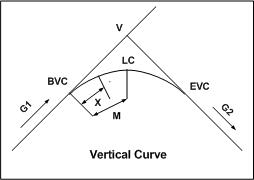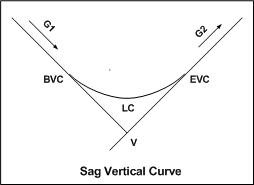* Log In to use the Calculate function * Become a Member!

Calculate The Vertical Curve Point Elevation:

Calculation:
Designer/Checker:

Input:Rate of grade change (R - %/sta)
Distance to point on curve (X - sta.)
Grade going into vertical curve (G1 - %)
Beginning vertical curve elevation (ElBVC - ft or m)

* Enter ( + ) values for ascending grades and ( - ) for descending grades.Output: